# Program to check Palindrome Number In Java

In this tutorial you will learn about the Program to check Palindrome Number In Java and its application with practical example.

In this tutorial, we will learn to create a Java Program to Check Palindrome Number In Java using Java programming.

## Prerequisites

Before starting with this tutorial we assume that you are best aware of the following Java programming topics:

• Java Operators.
• Basic Input and Output function in Java.
• Class and Object in Java.
• Basic Java programming.
• If-else statements in Java.
• For loop in Java.

## What is Palindrome Number.

A number is called a  Palindrome Number  if number  is same after reverse the number it self.

121 <=> 121

323 <=> 323

656 <=>656.

### Algorithm  to find Palindrome Number

1. Get the number from user to check  condition for palindrome
2. pass  the number in temporary variable.
3. Reverse the number.
4. Check  Temporary number with reversed number.
5. If both numbers are same number is “Palindrome number”.
6. Else not palindrome.

## Program to check Palindrome Number In Java

In this program we would find given number is a Palindrome number or not .first of we would take a value from user and find the Palindrome number.let have a look at the code.

## Output

Palindrome number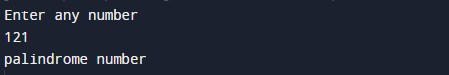Not a palindrome  Number.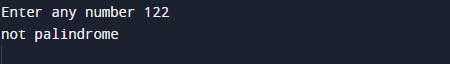In the above program, we have first declared and initialized a set variables required in the program.

• number= it will hold entered number.
• sum=   it will hold sum of factors.

After that we take a number from user and find given number is a Palindrome number or not.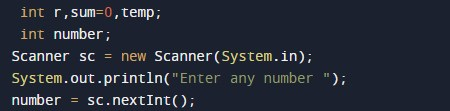Now we will run the loop until (number>0)

• Here we used a while loop to reverse number and store the reversed number in sum.
• Then we will check entered number is equal to the reverse number.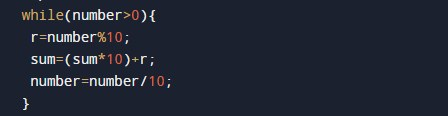• If Number is equal to reverse number (number==sum) then number is palindrome.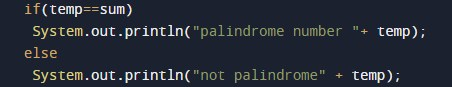If not number is not a Palindrome.

In this tutorial we have learn about the Program to check Palindrome Number In Java and its application with practical example. I hope you will like this tutorial.